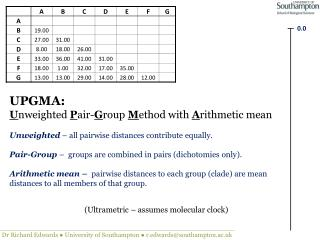DownloadDownload Presentation0.0

# 0.0

Télécharger la présentation## 0.0

- - - - - - - - - - - - - - - - - - - - - - - - - - - E N D - - - - - - - - - - - - - - - - - - - - - - - - - - -
##### Presentation Transcript

1. 0.0 UPGMA:Unweighted Pair-Group Method with Arithmetic mean Unweighted – all pairwise distances contribute equally. Pair-Group– groups are combined in pairs (dichotomies only). Arithmetic mean – pairwise distances to each group (clade) are mean distances to all members of that group. (Ultrametric – assumes molecular clock) Dr Richard Edwards ● University of Southampton ● r.edwards@southampton.ac.uk

2. B F 0.0 0.5 0.5 0.5 1.0 / 2 0.5 + 0.5 = 1.0 1. Find the shortest pairwise distance. 2. Join two sequences/groups with shortest distance. 3. Depth of new branch = ½ shortest distance. 4. Tip-to-tip path length = shortest distance.

3. B F 0.0 0.5 0.5 0.5 5. Calculate mean pairwise distances with other sequences in new matrix. (19 + 18) / 2 = 18.5 A (31 + 32) / 2 = 31.5 C B F D E G (18 + 17) / 2 = 17.5 (36 + 35) / 2 = 35.5 (13 + 12) / 2 = 12.5

4. A D B F 0.0 0.5 0.5 0.5 4.0 4.0 4.0 4.0 + 4.0 = 8.0 8.0 / 2 6. Repeat cycle with new shortest distance.

5. A D B F 0.0 0.5 0.5 0.5 4.0 4.0 4.0

6. A D B F 0.0 0.5 0.5 0.5 4.0 4.0 4.0 (19 + 18 + 18 + 17) / 4 = 18.0 C A B D F E G (27 + 26) / 2 = 26.5 (33 + 31) / 2 = 32.0 (13 + 14) / 2 = 13.5

7. A D B F G 0.0 0.5 0.5 0.5 4.0 4.0 6.25 5.75 4.0 6.25 12.5 / 2 0.5 + 5.75 + 6.25 = 12.5

8. A D B F G 0.0 0.5 0.5 0.5 4.0 4.0 6.25 5.75 4.0 (19 + 18 + 13 + 18 + 17 + 14) / 6 = 16.5 6.25 C A B D F E G New distances are mean values for all possible pairwise distances between groups.

9. A D B F G 0.0 0.5 0.5 0.5 4.0 4.0 6.25 5.75 4.0 (19 + 18 + 13 + 18 + 17 + 14) / 6 = 16.5 6.25 C A B D F E G (31 + 32 + 29) / 3 = 30.67 (36 + 35 + 28) / 3 = 33.0

10. A D B F G 0.0 0.5 0.5 0.5 4.0 4.0 6.25 5.75 4.0 4.25 6.25 2.0 8.25 16.5 / 2 0.5 + 5.75 + 4.25 = 16.5 4.0 + 4.25 + 6.25 + 2.0 = 16.5

11. A D B F G 0.0 0.5 0.5 0.5 4.0 4.0 6.25 5.75 4.0 (27 + 31 + 26 + 32 + 29) / 5 = 29.00 4.25 6.25 2.0 8.25 (33 + 36 + 31 + 35 + 28) / 5 = 32.60

12. A D B F G C 0.0 0.5 0.5 0.5 4.0 4.0 6.25 5.75 4.0 4.25 6.25 2.0 8.25 14.5 5.25 14.5 29.0 / 2

13. A D B F G C 0.0 0.5 0.5 0.5 4.0 4.0 6.25 5.75 4.0 (33 + 36 + 41 +31 + 35 + 28) / 6 = 34.00 4.25 6.25 2.0 8.25 14.5 5.25 14.5

14. A D B F G C E 0.0 0.5 0.5 0.5 4.0 4.0 6.25 5.75 4.0 4.25 6.25 2.0 8.25 14.5 5.25 UPGMA assumes a molecular clock. The tree is rooted with the final joining of clades. All tip-to-tip distances via the root will have the same total distance, equal to the final mean distance. 14.5 17.0 2.5 17.0

15. A D B F G C E 0.0 0.5 0.5 0.5 4.0 4.0 6.25 5.75 4.0 4.25 6.25 2.0 8.25 14.5 5.25 14.5 17.0 2.5 17.0

16. A D B F G C E 0.0 0.5 0.5 0.5 4.0 4.0 6.25 5.75 4.0 The source data for this worked example is a selection of Cytochrome C distances from Table 3 of one of the seminal phylogenetic papers: Fitch WM & Margoliash E (1967). Construction of phylogenetic trees. Science155:279-84. 4.25 6.25 2.0 8.25 http://www.ncbi.nlm.nih.gov/pubmed/5334057 14.5 5.25 14.5 17.0 2.5 17.0

17. Vertebrates Amniota 0.0 0.5 Primates 4.0 6.25 8.25 14.5 17.0 Reptilia Mammals Turtle Chicken Man Monkey Dog Tuna Moth The UPGMA tree based on this Cytochrome C data supports the known evolutionary relationships of these organisms.